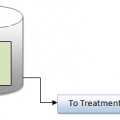## Food-to-Microorganism Ratio(F/M Ratio)

Food-to-Microorganism ratio (F/M ratio)

The food-to-microorganism ratio (F/M ratio) is a process control method/calculation based upon maintaining a specified balance between available food materials (BOD or COD) in the aeration tank influent and the aeration tank mixed liquor volatile suspended solids (MLVSS) concentration The chemical oxygen demand test is sometimes used, because the results are available in a relatively short period of time. To calculate the F/M ratio, the following information is required:

• Aeration tank influent flow rate (MGD)
• Aeration tank influent BOD or COD (mg/L)
• Aeration tank MLVSS (mg/L)
• Aeration tank volume (MG)

F/M ratio=  primary effluent COD/BOD (mg/L)  x Flow (MGD) x 8.34 lb/mg/L/MG /MLVSS (mg/L) x aerator volume (MG) x 8.34 lb/mg/L/MG
Example:-

The aeration tank influent BOD is 145 mg/L, and the aeration tank influent flow rate is 1.6 MGD.What is the F/M ratio if the MLVSS is 2300 mg/L and the aeration tank volume is 1.8 MG?

F / M ratio= 145mg/L x1.6 MGD x 8.34 lb/mg/L /MG
2300 mg /L x1.8 MG x 8.34 lb/mg/L/MG
= 0.06 BOD (lb) / MLVSS (lb)

1. Key Point:
If the MLVSS concentration is not available, it can be calculated if the percent volatile matter (%VM) of the mixed liquor suspended solids (MLSS) is known.
MLVSS = MLSS x % (decimal) volatile matter (VM)
2. Key Point:
The food (F) value in the F/M ratio for computing loading to an activated biosolids process can be either BOD or COD. Remember, the reason for biosolids production in the activated biosolids process is to convert BOD to bacteria. One advantage of using COD over BOD for analysis of organic load is that COD is more accurate.## Author:T.P.Sivanandan

#### Why Fine Bubbles are Better than Coarse bubble in Aeration Tank#### How to Calculate Lime Dosage Requirement in Sedimentation Process## 5 thoughts on “Food-to-Microorganism Ratio(F/M Ratio)”

1.Urvish rathod says:

Our aeration tank cod 700 to 900 mg/lit ,aeration flow rate 70 kl/day ,aeration tank vol 500 kl,and mlss 6000 mg/lit or mlvss 3600 mg/lit ,so find how much our f/m ratio

1.Manhar says:

0.311

1.Satheesh Kumar says:

Pls could you tell determine that calculation

2.Y. DCosta says:

Our BOD is 40 mg/ Lt, flow is 6.0 MLD, MLVSS is 1200, and vol of aeration tank is 2020 cubic metres.Please calculate F/ M ratio. Thanks

1.Pramod Pisal says:

The F/M ratio is 0.44 as below;

40(mg/l) x 6 ( MLD ) X 8.34 ( lb/mg/l/MG) / 1200 ( mg/l ) x 0.4445 ( MG ) x 8.34 ( lb/mg/l/MG)

F/ M Ratio = 0.44